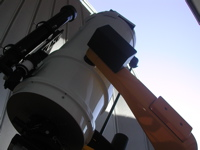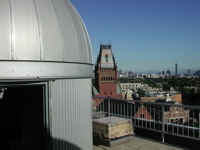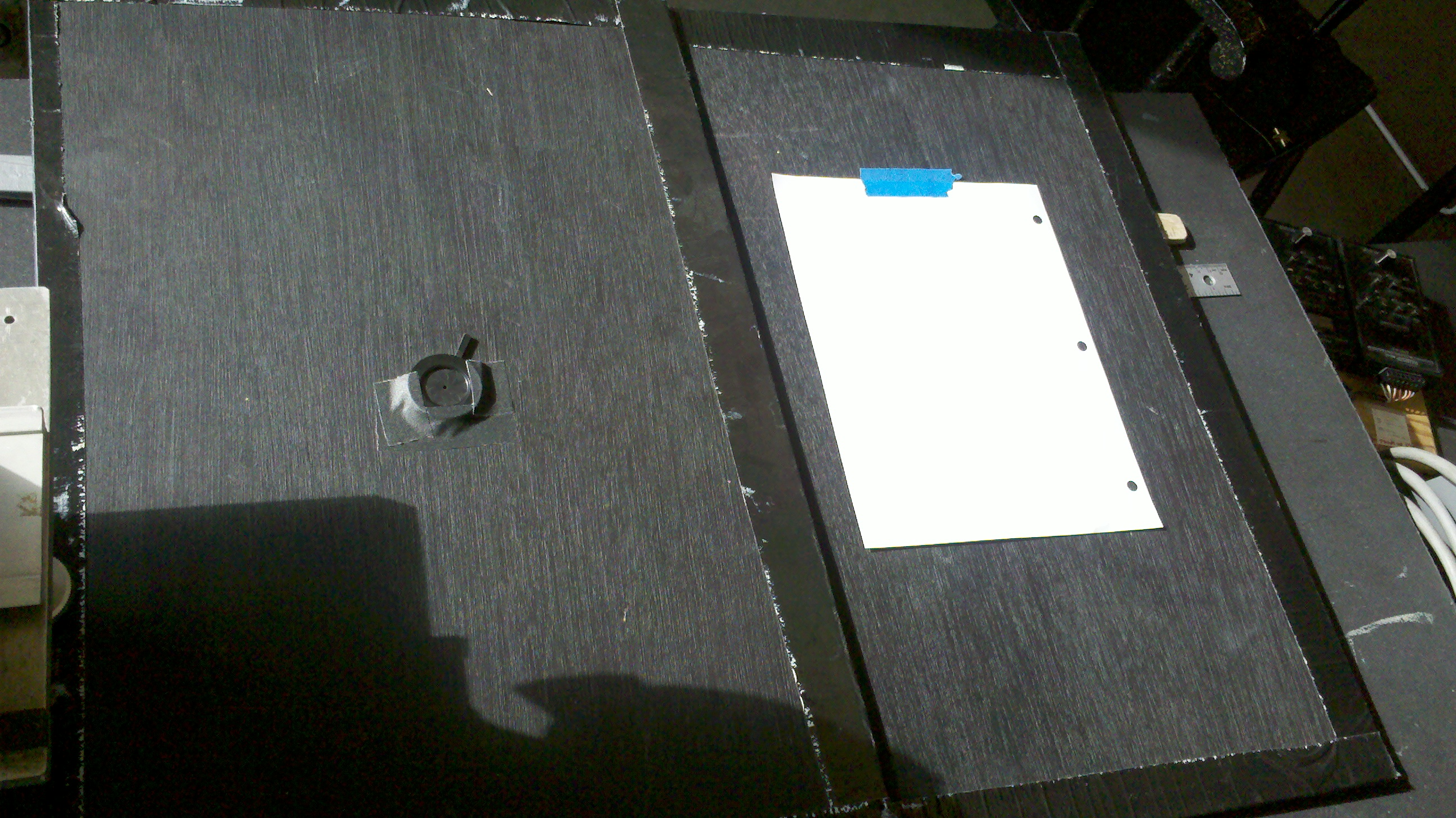# Astronomy Lab and Clay TelescopeMeasurement of the Sun’s Diameter using the Pinhole

SPU-21 Fall 2015

printer version

The physical diameter of a star is fundamental for its temperature, luminosity (energy output), and other important properties. It is easy to determine the diameter of the Sun if we know its distance, the Astronomical Unit (AU), which is approximately 1AU = 1.5x 108 km. The method we will use to measure the angular diameter of the Sun is simple geometry.

What you'll need:• 2 or more students
• white sheet of paper
• pencil
• tape
• the pinhole boards [16” x 24”], one solid and one with an iris
• meter measuring stick

Procedure:

• Tape a white sheet of paper to the solid black board without the iris.
• Lean the board with the paper taped to it against a concrete block on the 8th floor deck so that it is approximately perpendicular to the direction of the Sun.
• One student will hold the black board with the iris parallel to the paper and perpendicular to the Sun's direction at the distance of the meter stick.
• The sunlight shines through the iris (which is adjustable) and creates a small round spot of light on the paper. Adjust the iris so the sun is in focus, giving a sharp image.
• Take turns tracing the spot on the paper and then measuring the spot. Make sure to take at least 3-5 measurements!

Analysis:

• The ratio of the diameter of the spot of light to its distance to the pinhole is just the ratio of the actual diameter of the Sun, to the distance from the Sun to the lens, which is approximately 1 AU = 1.5 x 108 km.
• Dimage/dimage = Dsun/ 1.5x108 km, where D is the diameter and d is the distance (be consistent with units and use cm to measure the image diameter!)
• Take at least 3-5 measurements of each quantity: the diameter from top to bottom, Dup-down, the diameter from left to right, Dleft-right, and the distance from the iris to the sheet of paper, d. Enter these measurements into the table (pdf or docx).
• Then determine the averages and estimate the uncertainty (see Handout - Error Measurements).
• Your answer will not be exactly correct, of course, because of inevitable measurement errors, or uncertainties in your measurements, which are vital to consider and report in your Observation Summary.
• Finally, we will calculate the Luminosity of the Sun using the blackbody equation (Stefan-Boltzmann Law).
• L = 4πR2σT4
• L is the luminosity of the Sun in Watts, R is the radius of the Sun in meters, σ is a constant of physics known as the Stefan-Boltzmann constant (σ = 5.67 x 10-8 watt/m2/degree4), and T is the surface temperature of the Sun in degrees Kelvin (degrees above absolute zero).
• Given that the Sun's effective temperature is 5780 K and the fact that you found the Sun's diameter above, you can now use the Stefan-Boltzmann Law to calculate the Sun's Luminosity.